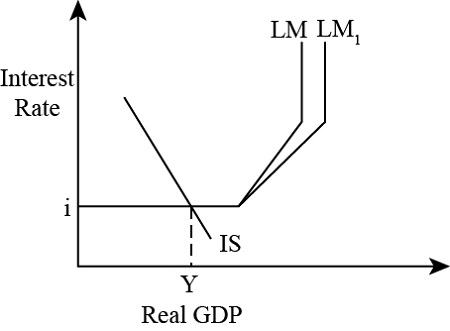# What happens to the LM curve during a liquidity trap, where interest rates are sufficiently...

## Question:

What happens to the LM curve during a liquidity trap, where interest rates are sufficiently negative to the point that they surpass the cost of holding cash? Would it cease to continue horizontally, as it is impossible to increase GDP through monetrary policy? Explain through a graph.

## Monetary policy and LM curve :

The monetary policy is the policy of the central bank of the country, and it is used to control the economic conditions of the economy at the time of recession or inflation. The LM curve is an upward sloping curve that shows the positive relationship between the interest rate and real GDP where the money market is in equilibrium.

## Answer and Explanation:

The liquidity trap is a situation when the interest rate in the economy is too low or zero that cannot be decreased further to increase output in the economy. In this case, increment in the money supply does not reduce the interest rate because the interest rate is already extremely low. As a result, the money demand and the LM curve become horizontal, and the LM curve does not shift due to increment in the money supply. Hence, the monetary policy is ineffective in case of a liquidity trap. The monetary policy is effective when the LM curve is upward sloping.

The situation of the liquidity trap is given below:When the money supply is increased, the vertical and upward sloping part of the LM curve shifts rightward, but the horizontal section of LM does not shift. Hence, monetary policy is ineffective.

#### Learn more about this topic:LM Curve in Macroeconomics: Definition & Equation

from Introduction to Business: Homework Help Resource

Chapter 3 / Lesson 46
36K